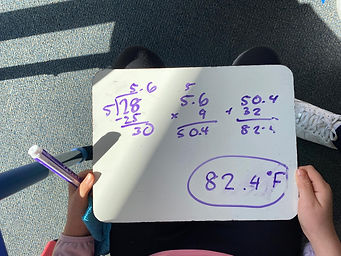Mr. Jake

Target 1​

Lesson Type:

Continuation

Measurment

:

Unit Conversion

Convert measurement data between measurement systems.

1:

Describe the relationship between units that are being converted.

2:

Explain how measurement units differ or are similar.

3:

Explain why the value of a measurement is not changing (only the unit) when using a conversion factor.

4:

Use ratio understandings to convert measurement units.

5:

Solve application problems involving the conversion of measurement units.

7th

Vocabulary:

Unit, Convert

Activities:

Practiced converting between different units, including fractional values

Used the formula for converting Fahrenheit to Celsuis, and determined how to reverae the formula to convert Celsius to FahrenheitHome Exploration

Guiding Questions:Absent Students:

Target 2

:

Vocabulary:

Activities:Home Exploration

Guiding Questions:Target 3

:

Vocabulary:

Activities:Home Exploration